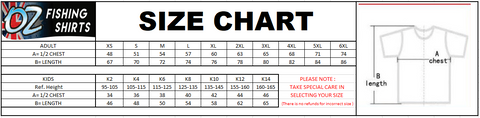# SIZE CHART - in cm

\$0.00
Product descriptionMeasurements  are in cm

we recommend for ladies

8= XS, 10= SML, 12= M, 14= L,  16= XL, 18, = 2XL, 20= 3XL, 22= 4XL, 24= 5XL

26= 6XL, 28= 7XL, 30= 8XL